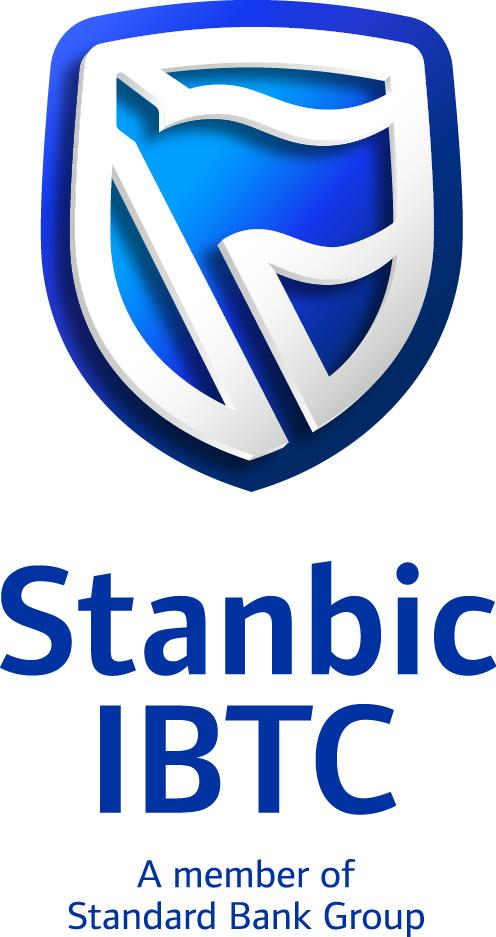Trending Today#### Stanbic IBTC Holdings: Juicier Interim Dividend, Despite Weaker EarningsRating: Hold
Current Market Price: N38.90
Loan to Deposit Ratio: 56.84%
Year High: N53.25
Year Low: N38.70
Fair Value: N46.66
Equity AnalystTunde Segun Jeariogbe

Introduction

• This report observes the financial performance of Stanbic IBTC Holdings Plc for the half-year ended 30th June 2021, compares same with that of the similar period in 2020 to establish growth path.
• We also observed few valuation metrics and achieved conservative projections using the full-year performance indices over the past few years.
• We have observed that over the years, the interim dividend is always higher than the final payout, and so, we have followed the same trend in our valuation projections.
• Although the board of Stanbic IBTC announced a higher interim dividend of N1.00 per share, up from the previously N0.40 each, despite the fact that the financial came far below what was reported in the corresponding period of 2020.
• See below for other financial indices:

Company figures

• Gross Earnings for the period stood at N93.59 billion, 26.06% down from the N126.57 billion posted at the end of 2020 half-year.
• Interest Income stood at N44.22 billion down from the N55.13 billion in 2020 first half.
• Interest Expenses was estimated at N11.35 billion, same as 35.44% below the reported N17.58 billion at the end of the corresponding period of 2020.
• Net Interest Income was estimated at N32.87 billion from the N37.54 billion achieved last half-year.
• Operating Expenses on the other hand, grew by 14.07% above the comparable period, to N55.36 billion from N48.53 billion.
• Profit before Tax stood at N24.70 billion, a 52.85% drop from the N54.06 billion posted in the 2020 half-year.
• After considering the Tax Expense for the period, a total of N22.54 billion was reported as Profit for the period, which is 50.13% below the N45.20 billion earned at the end of the first six months in 2020.
• Total Comprehensive Income for the period stood at N8.45 billion, as against N56.02 billion in the comparable period of 2020.
• Total Assets is currently valued at N2.42 trillion, same as 19.68% below that of the 2020 full-year at N3.02 trillion.
• Similarly, the Total Liabilities posted for the period was N2.08 trillion, against N2.68 trillion in 2020.
• Net Assets is therefore estimated at N346.44 billion, or 2.95% above the N336.52 billion estimated in the corresponding period of 2020.
• Retained Earnings dipped by a marginal 2.76% to N230.09 billion from N236.62 billion in the comparable half-year.
• Total Deposit at the end of the period is valued at N1.35 trillion, higher than the N1.21 trillion deposit reported in the corresponding first half of 2020.
• Total Loans and Advances stood at N767.81 billion, against N579.48 billion reported in the similar period of 2020.

Financial Strength/Solvency Ratios

• Debt Ratio is estimated at 85.73% from 88.87% in the corresponding period of 2020. In other words, the Total Liability Value Reported is currently 85.73% of Total Assets Valuation.
• Total Debt to Equity for the quarter stood at 601%, which means that Debt can Replicate Equity 6.01 times, as against 7.98 times replicate in the comparable period.
• Equity Ratio which compares Equity to Total Assets stood at 14.27%, higher than the 11.13% reported in the corresponding period of last year.
• Estimated Beta value for Stanbic IBTC Bank as at the time this report was compiled stood at 1.15x, which although is above the market beta, stood above the industry average 1.11x.

Profitability Ratios

• Pre-Tax Margin dropped by 36.24% to 26.40%, against the 41.40% estimated at the end of 2020 half-year.
• Interest Expenses to Gross Earnings dropped by 12.69% to 12.13% from the previously estimated 13.89% in the corresponding quarter.
• Return on Average Equity through the first six months of 2021 is 6.51%, against 13.43% estimated from the 2020 numbers.
• See the table below for detailed profitability ratios and comparisons.

Efficiency Ratios

• Operating Expenses at the end of the period is estimated at 59.16% of the estimated Gross Earning, which is above the 38.35% achieved in the 2020 six-month financials.
• Gross Earnings estimated from the financials is the same as 3.86% of the Total Assets value posted for the period under analysis.
• Total Loan and Advances posted for the quarter is 56.84% of Total Deposit, which is a 19.56% upward adjustment over the 47.54% achieved in 2020. Note that this is below the regulatory threshold for Nigerian banks.

Investment/Valuation Ratios

• The profit earned per unit of Stanbic IBTC Holdings’ shares is estimated at N2.15 each, a  50.13% below the N4.30 earned in a similar half-year of 2020.
• Total Comprehensive Income is estimated at N0.81, about 84.90% above the N5.33 TCI/share achieved in 2020.
• Our adjusted P/E-Ratio for six months dropped to 4.57x from 2.18x estimated at the end of 2020 half year business session.
• The said earnings per share yielded 5.47% of the price of each unit of
Stanbic IBTC Bank’s shares on the exchange on the day the result was unveiled to investors through the exchange
• Estimated value of each unit of Stanbic IBTC shares in its book is N32.98, this is 2.95% improvement over the previous estimate of N32.03 per share.
• Confirming the academic over-priced status of Stanbic IBTC shares on the ‘’’exchange is the Price to Book Value estimate at above unity.

Valuation

Exploring our blend of valuation tools, we conservatively valued each unit of Stanbic IBTC shares at N46.66 per share. Thus, considering the mild difference between our intrinsic value and the current market price as at the time this analysis was compiled, we rated Stanbic IBTC shares a Hold.